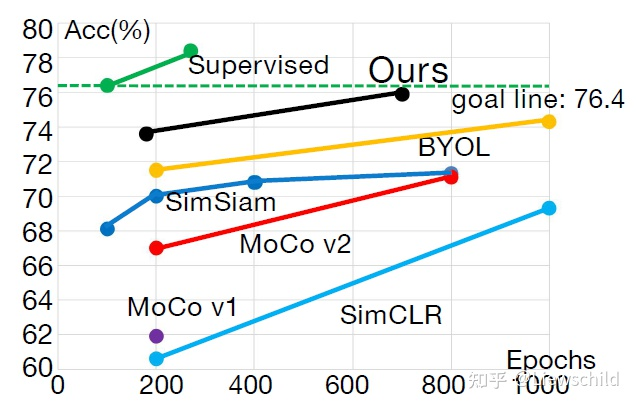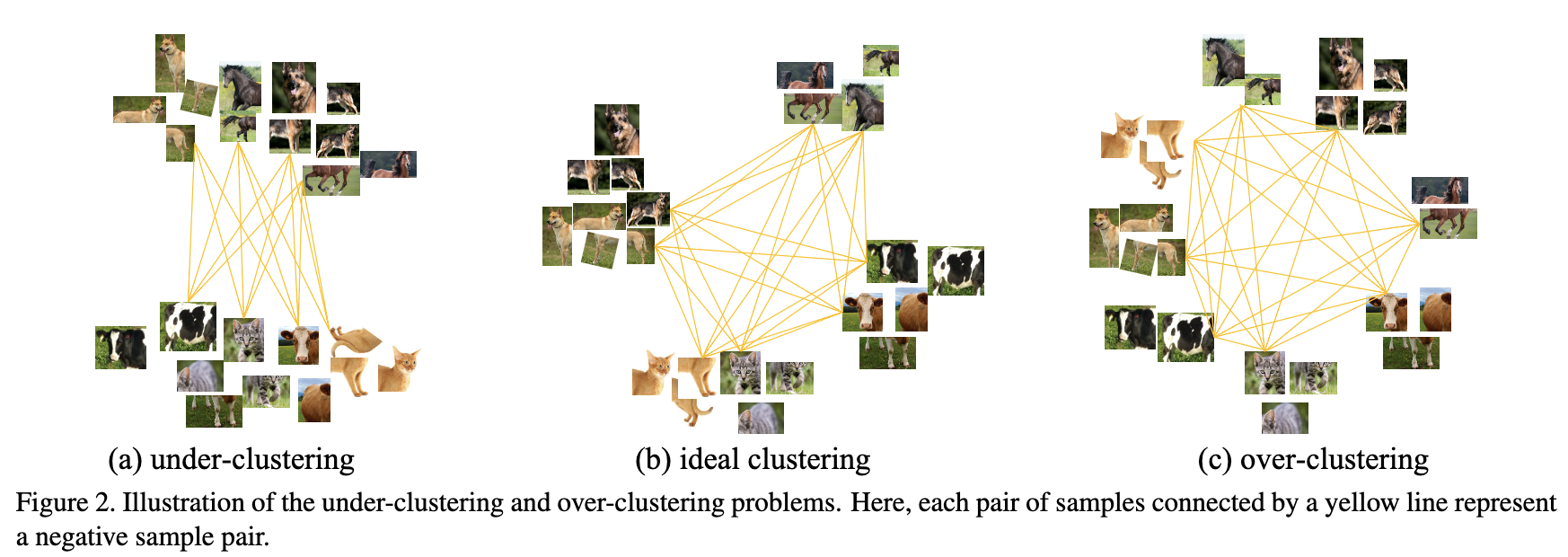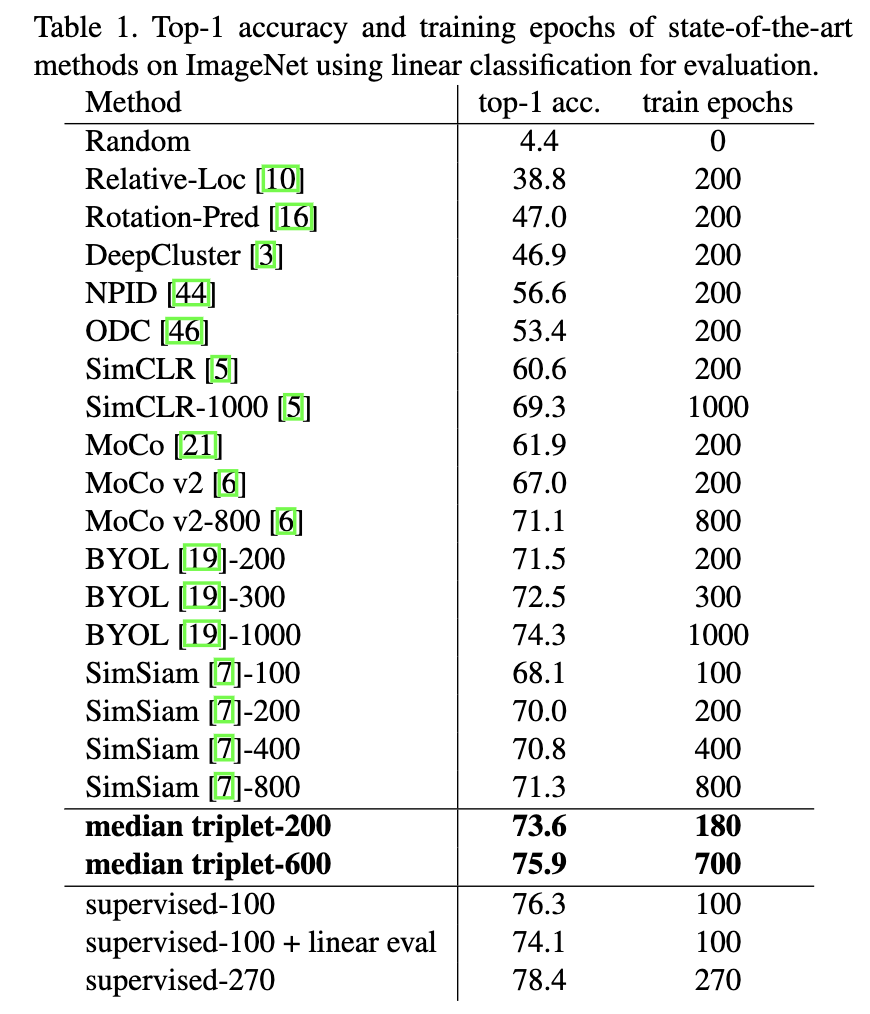Solving Inefficiency of Self-supervised Representation Learning

IntroductionUnder-clustering and Over-clustering ProblemsMedian Triplet Loss

$$\mathcal{L o s s}=\max \left(d\left(x, x^{+}\right)-d\left(x, x_{\text {hardest }}^{-}\right), \mathcal{C}\right)$$

$$\mathcal{L o s s}=\max \left(\gamma d\left(x, x^{+}\right)-d\left(x, x_{\text {rank-k }}^{-}\right), \mathcal{C}\right)$$

Main Results